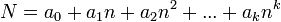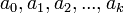# Big numbers

Big numbers, known colloquially as bignums (adjectival form bignum, as in bignum arithmetic) are integers whose range exceeds those of machine registers. For example, most modern processors possess 64-bit registers which can be used to store integers up to 264-1. It is usually possible to add, subtract, multiply, or divide such integers in a single machine instruction. However, such machines possess no native implementation of arithmetic on numbers larger than this, nor any native means of representing them. In some applications, it might be necessary to work with numbers with hundreds or even thousands of digits.

Of course, humans have no problems with working with numbers greater than 264-1, other than the fact that it becomes increasingly tedious as the numbers grow larger; we just write them out and use the same algorithms we use on smaller numbers: add column-by-column and carry, and so on. This turns out to be the key to working with bignums in computer programs too. When we write out a number in decimal representation, we are essentially expressing it as a string or array of digits and the algorithms we use to perform arithmetic on them, which entail examining the digits in a particular order, may be considered as loops.

## Nonnegative integers

### Digital representation

Most of the time, and almost all of the time in contests, the only bignums the programmer will be required to implement are positive integers. As alluded to above, bignums in computer programs are represented using a radix system. This means that a bignum is stored in a base$n$ representation, where the choice of$n$ is based on the application. Precisely, the nonnegative integer$N = a_0 + a_1 n + a_2 n^2 + ... + a_k n^k$ is stored by giving the values$a_0, a_1, a_2, ..., a_k$, which we probably want to store as an array. Here are a few common possibilities, demonstrated for the example of 30! = 265252859812191058636308480000000:

• If we let the radix be 10:
• ASCII representation: the number is represented by a string which literally contains the number's digits as characters: 265252859812191058636308480000000 would be represented ['2','6','5',...,'0']
• BCD representation: an array of digits (not their ASCII values), hence [2,6,5,...,0]
• If we let the radix be 109, we can store nine digits in each array entry. For example, 30! could be stored [265252,859812191,058636308,480000000]. Note that we must take care to group digits starting from the ones' place and moving left, instead of starting at the most significant digit and moving right, to avoid complicating the code and eroding performance. This is because adding and subtracting bignums requires that they be aligned at the decimal point (not at the most significant digit), so it is convenient when we guarantee that this is never found within an entry, otherwise shifting would be necessary.
• If we let the radix be 232, we use a sequence of 32-bit unsigned integer typed variables to store the bignum. We know 26525285981219105863630848000000010 = D13F6370F96865DF5DD5400000016. Again, we align at the ones' place, giving [00000D1316,F6370F9616,865DF5DD16,5400000016]. (Of course, you should not actually explicitly store the hex representations, because that would be cumbersome and slow. It's just an array of 32-bit values.)

### Little-endian vs. big-endian

The byte is the fundamental addressable unit of memory for a given processor. This is distinct from the word, which is the natural unit of data for a given processor. For example, the Intel 386 processor had 8 bits to a byte but 32 bits to a word. Each byte in memory may be addressed individually by a pointer, but one cannot address the individual bits in them. That being said, when a 32-bit machine word is written to memory, there are two ways it could be done. Suppose the number CAFEBABE16 is stored at memory location DEADBEEF16. This will occupy four bytes of memory, and they must be contiguous so that the processor can read and write them as units. The important question is whether the most significant byte (in this case CA16) comes first (big-endian) or last (little-endian). The following table shows where each byte ends up in each scheme.

Big-endian CA16 FE16 BA16 BE16
Little-endian BE16 BA16 FE16 CA16

One faces a similar choice when storing bignums: does the most significant part get stored in the first or the last position of the array? Almost every processor is either consistently little-endian or consistently big-endian, but this does not affect the programmer's ability to choose either little-endian or big-endian representations for bignums as the application requires. The importance of this is discussed in the next section.

### Fixed-width versus dynamic-width bignums

There are, in principle, two kinds of bignum implementation. Suppose we know in advance the maximum size of the integers we might be working with. For example, in [email protected], we are asked to report the number of permutations which satisfy a certain property. There are only up to 30 elements, so we know that the answer will not exceed 30! = 265252859812191058636308480000000, which has 33 digits. It is not terribly difficult to implement the solution in such a way that no intermediate variable is ever larger than this. So we could, for example, use a string of length 33 to store all integers used in the computation of the answer (where numbers with fewer than 33 digits are padded with zeroes on the left), and treat all numbers as though they had 33 digits. The only time when we would care how many digits the number actually has is when outputting it. Addition can now be implemented as a loop over 33 columns, and is fairly simple. This implementation is called fixed-width.

On the other hand, sometimes it is not so easy to determine in advance the size of the numbers we might be working with, or a problem might have bundled test cases and a strict time limit, forcing the programmer to make the small cases run more quickly than the large ones. When this occurs it is a better idea to use dynamic bignums, which can expand or shrink according to their length. Dynamic bignums are trickier to code than fixed-width ones: when we add them, for example, we have to take into account that they might not be of the same length; we might then treat all the missing digits as zeroes, but in any case it requires extra code. When using dynamic bignums the difference between the little-endian and big-endian representations becomes significant. If we store the bignums little-endian, and add them, alignment is free: just look at the first entry in each of them; they are in the ones' places of their respective numbers. The code presented in this article will assume the little-endian representation.

### Operations

This section describes how to actually manipulate bignums. We assume a dynamic zero-based little endian array representation but leave lots of details to the programmer.

The schoolbook addition algorithm first adds the ones places, then adds the tens (with a carry, if necessary), then the hundreds, and so on. We will likewise start by adding the ones places and proceed to more significant digits.

```input bignums x,y
n ← length of x
m ← length of y
p ← max(m,n)
carry ← 0
for i ∈ [0..p)
carry ← 1
else
carry ← 0
if carry
z[p] ← 1
p ← p+1
```

After this code has completed, `z` will hold the sum of `x` and `y`, and `p` will be the length of `z` (the number of nonzero places). There are two caveats, though:

• When adding bignums of equal length, the loop counter `i` will grow beyond the length of one of the two. What does `x[i]` mean when `i``n`? To make this code work, it should be treated as zero. In a working implementation, we must take care to avoid out-of-bounds array access.
• If the radix used is the same size as a machine word, then we cannot actually check whether `x[i]+y[i]+carry ≥ radix` as shown. Instead, check whether the result is greater that or equal to both `x` and `y`. If it is not, an overflow has occurred (and the carry bit should be set.)

Nevertheless, this shows the basic idea behind the addition of bignums. Here is a sample of C++ code as it might actually appear, using radix 10:

```// x, y, and z are vectors of digits
{
int n = x.length();
int m = y.length();
int p = max(n,m);
z.resize(p);
int carry = 0;
for (int i=0; i<p; i++)
{
int t=carry;
if (i<n) t+=x[i];
if (i<m) t+=y[i];
z[i]=t%10;
carry=t/10;
}
if (carry)
z.push_back(1);
}
```

### Error conditions

What happens if the program tries to divide a bignum by zero? In almost all popular programming languages (but not C), one can take advantage of built-in-support for exceptions. If the bignum division function raises an exception, this can be caught by the calling function and handled somehow. What we do not want is an unconditional crash of the program. There are also some other conditions that can generate unpredictable behavior, such as integer overflow. It is important to check for these in the code and take appropriate action (such as raising an exception).

• Check that you don't divide by zero.
• Check that a larger number is not subtracted from a smaller number, otherwise unpredictable behavior may result.
• Fixed-width bignums may overflow when adding or multiplying. You will be able to detect this because a carry is generated in the left-most column when adding, or something like that. Naively failing to check this may result in out-of-bounds array access.
• Check that the input does not overflow a fixed-width bignum. Naively failing to check this will probably result in an out-of-bounds array access.
• If you're writing a bignum library, and intend to reuse the code, you might also want to check, with dynamic bignums, that your attempts to allocate more memory actually succeed. (You might be sure that your computer and the judge computer have enough memory for the purposes of this particular problem, but maybe you'll do another problem later with a lower memory limit, or someone else with less RAM will use your code.) If not, and you just keep going on anyway, a segfault is almost certain to occur. If you do check, you can throw an exception.